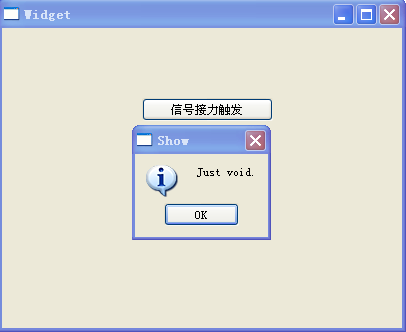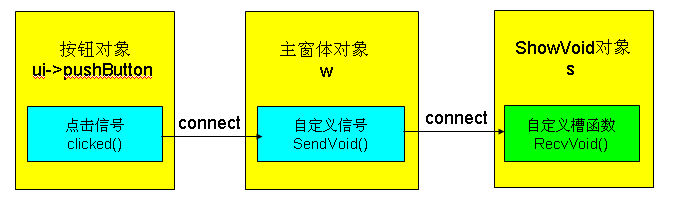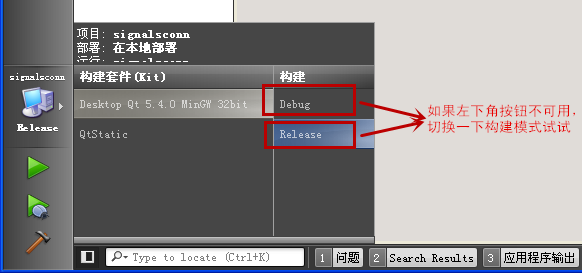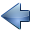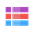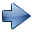4.3 自定义信号和槽

4.3.1 C++ 的沟通方式

C++ 编程中常遇到各个对象之间进行沟通的情景，需要将数据从一个对象传递给另一个对象来处理。大致的方法有如下几种：
• 接收端定义公有成员变量以供源端修改，然后接收端处理数据； （不建议用！
• 接收端将私有成员变量通过友元方式共享给源端，源端可以修改接收端变量； （除了特殊情况，一般不建议用！
• 接收端定义公开的 get 和 set 函数，提供给源端调用 （推荐使用，可以与信号和槽机制协同工作）；
• 源端给出回调函数约定，接收端定义相同参数和返回值类型的静态成员函数，将静态成员函数作为回调函数交给源端，源端再调用该函数 （可以使用）。

//publicmember.cpp
`#include <iostream>`
`using namespace std;`
`//接收端类`
`class Dst`
`{`
`public:`
`    double m_dblValue;`
`    int m_nCount;`

`    //处理数据函数`
`    double DoSomething()`
`    {`
`        double dblResult = m_dblValue / m_nCount;`
`        return dblResult;`
`    }`
`};`
`//源端类`
`class Src`
`{`
`public:`
`    void SendDataTo( Dst &theDst)`
`    {`
`        //设置接收端公有变量`
`        theDst.m_dblValue = 2.0;`
`        theDst.m_nCount = 3;`
`    }`
`};`

`int main()`
`{`
`    //定义两个对象`
`    Dst theDst;`
`    Src theSrc;`
`    //传递数据`
`    theSrc.SendDataTo(theDst);`
`    //接收端处理`
`    cout<<theDst.DoSomething();`
`    //`
`    return 0;`
`}`

//friend.cpp
`#include <iostream>`
`using namespace std;`

`//接收端类`
`class Dst`
`{`
`private:    //私有变量`
`    double m_dblValue;`
`    int m_nCount;`

`public:`
`    //处理数据函数`
`    double DoSomething()`
`    {`
`        double dblResult = m_dblValue / m_nCount;`
`        return dblResult;`
`    }`
`    //友元类`
`    friend class Src;`
`};`
`//源端类`
`class Src`
`{`
`public:`
`    void SendDataTo( Dst &theDst)`
`    {`
`        //因为是友元类，所以能设置接收端私有变量`
`        theDst.m_dblValue = 2.0;`
`        theDst.m_nCount = 3;`
`    }`
`};`

`int main()`
`{`
`    //定义两个对象`
`    Dst theDst;`
`    Src theSrc;`
`    //传递数据`
`    theSrc.SendDataTo(theDst);`
`    //接收端处理`
`    cout<<theDst.DoSomething();`
`    //`
`    return 0;`
`}`

//getset.cpp
`#include <iostream>`
`using namespace std;`

`//接收端类`
`class Dst`
`{`
`private:    //私有变量`
`    double m_dblValue;`
`    int m_nCount;`

`public:`
`    //get函数`
`    double value()`
`    {`
`        return m_dblValue;`
`    }`
`    int count()`
`    {`
`        return m_nCount;`
`    }`
`    //set函数`
`    void setValue(double v)`
`    {`
`        m_dblValue = v;`
`    }`
`    void setCount(int n)`
`    {`
`        if( n < 1 ) //防止除 0 ，并且计数限定为正整数`
`        {`
`            m_nCount = 1;`
`        }`
`        else`
`        {`
`            m_nCount = n;`
`        }`
`    }`

`    //处理数据函数`
`    double DoSomething()`
`    {`
`        double dblResult = m_dblValue / m_nCount;`
`        return dblResult;`
`    }`
`};`
`//源端类`
`class Src`
`{`
`public:`
`    void SendDataTo( Dst &theDst)`
`    {`
`        //通过set函数传递数据`
`        theDst.setValue(2.0);`
`        theDst.setCount(3);`
`    }`
`};`

`int main()`
`{`
`    //定义两个对象`
`    Dst theDst;`
`    Src theSrc;`
`    //传递数据`
`    theSrc.SendDataTo(theDst);`
`    //接收端处理`
`    cout<<theDst.DoSomething();`
`    //`
`    return 0;`
`}`

//callback.cpp
`#include <iostream>`
`using namespace std;`

`//源端约定回调函数类型`
`typedef void (*PFUNC)(double v, int n, void *pObject);`

`//接收端类`
`class Dst`
`{`
`private:`
`    double m_dblValue;`
`    int m_nCount;`

`public:`
`    //处理数据函数`
`    double DoSomething()`
`    {`
`        double dblResult = m_dblValue / m_nCount;`
`        return dblResult;`
`    }`
`    //回调函数`
`    static void FuncCallBack(double v, int n, void *pObject)`
`    {`
`        //转换成 Dst 指针`
`        Dst *pDst = (Dst *)pObject;`
`        //静态成员函数也是可以设置私有变量的，但需要手动传对象指针`
`        //设置 value`
`        pDst->m_dblValue = v;`
`        //设置count`
`        if( n < 1)`
`        {`
`            pDst->m_nCount = 1;`
`        }`
`        else`
`        {`
`            pDst->m_nCount = n;`
`        }`
`    }`

`};`
`//源端类`
`class Src`
`{`
`public:`
`    void SendDataTo( Dst *pDst, PFUNC pFunc)`
`    {`
`        //通过回调函数传数据`
`        pFunc(2.0, 3, pDst);`
`    }`
`};`

`int main()`
`{`
`    //定义两个对象`
`    Dst theDst;`
`    Src theSrc;`
`    //传递数据`
`    theSrc.SendDataTo(&theDst, Dst::FuncCallBack);`
`    //接收端处理`
`    cout<<theDst.DoSomething();`
`    //`
`    return 0;`
`}`

①theSrc 调用 SendDataTo 函数，参数有目标对象 theDst 指针和目标类里定义好的 回调函数 Dst::FuncCallBack。
②在 SendDataTo 函数内部，回调函数 Dst::FuncCallBack 作为 pFunc ，被调用执行，三个参数值为 2.0、3 和目标对象 指针。
③pFunc 就是 Dst::FuncCallBack 回调函数，这个回调函数会根据三个参数里数值，首先将 void * 指针转为目标对象指针 pDst，然后设置目标对象里的两个私有成员变量，并且可以直接做数据有效性检查。
④theDst 对象里的成员变量被设置好之后，就可以调用 DoSomething 函数做处理了。

4.3.2 通过自定义信号和槽沟通

• 源头只需要顾自己发信号就行，不用管谁会接收信号；
• 接收端只需要关联自己感兴趣的信号，其他的信号都不管；
• 只要源头发了信号，关联该信号的接收端全都会收到该信号，并执行相应的槽函数。

①项目名称 qobjcom，创建路径 D:\QtProjects\ch04，点击下一步；
②套件选择里面选择全部套件，点击下一步；
③基类选择 QWidget，点击下一步；
④项目管理不修改，点击完成。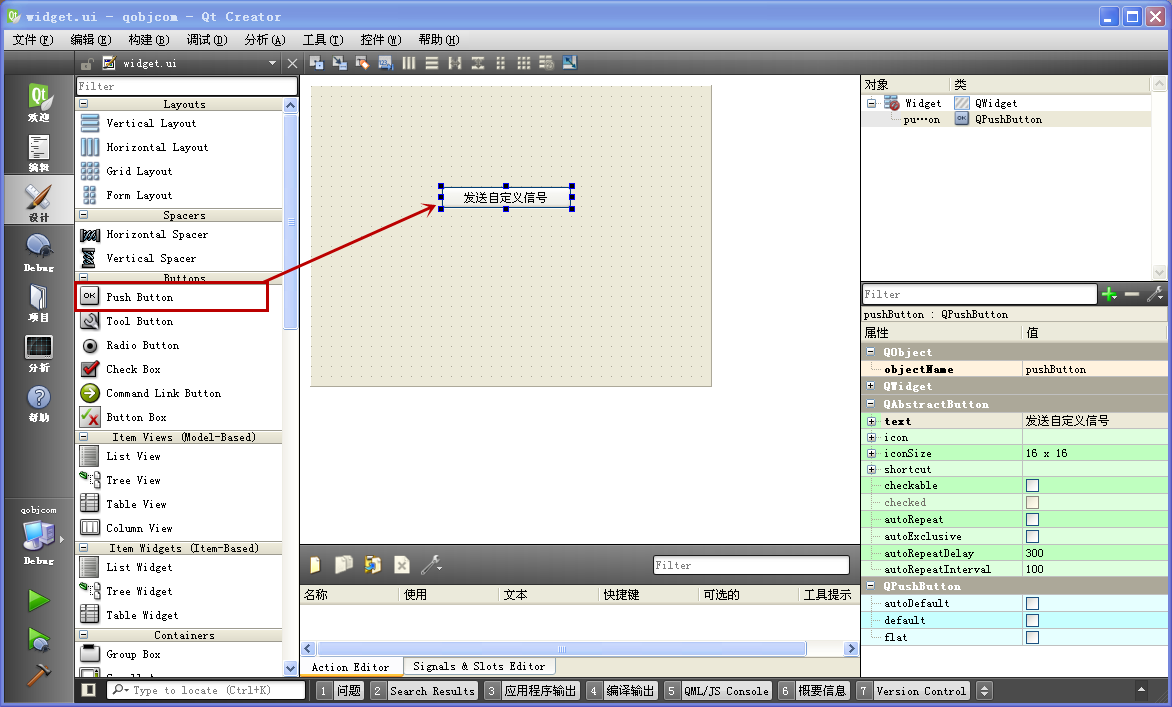#ifndef WIDGET_H
`#define WIDGET_H`

`#include <QWidget>`

`namespace Ui {`
`class Widget;`
`}`

`class Widget : public QWidget`
`{`
`    Q_OBJECT`

`public:`
`    explicit Widget(QWidget *parent = 0);`
`    ~Widget();`

`signals:    //添加自定义的信号`
`    void SendMsg(QString str);  //信号只需要声明，不要给信号写实体代码`

`public slots:   //接收按钮信号的槽函数`
`    void ButtonClicked();`

`private:`
`    Ui::Widget *ui;`
`};`

`#endif // WIDGET_H`
signals: 就是信号代码段的标志，这个标志不带 public 、protected、private 等前缀，那是因为信号默认强制规定为公有类型，这样才能保证其他对象能接收到信号。

public slots: 是公有槽函数代码段的标志，定义了 ButtonClicked 槽函数，接收按钮被点击的信号，这个槽函数以后会触发我们自定义的信号。槽函数代码段也只能放槽函数声明的代码，不要把其他的东西放在这个代码段里。

#include "widget.h"
`#include "ui_widget.h"`

`Widget::Widget(QWidget *parent) :`
`    QWidget(parent),`
`    ui(new Ui::Widget)`
`{`
`    ui->setupUi(this);`

`    //关联`
`    connect(ui->pushButton, SIGNAL(clicked()), this, SLOT(ButtonClicked()));`
`}`

`Widget::~Widget()`
`{`
`    delete ui;`
`}`
`//槽函数`
`void Widget::ButtonClicked()`
`{`
`    //用 emit 发信号`
`    emit SendMsg( tr("This is the message!") );`
`}`

ButtonClicked 里面只有一句代码，就是
emit SendMsg( tr("This is the message!") );
emit 是发信号的关键字，然后接下来就与调用函数是一样的格式，SendMsg 里面放置我们想传递的字符串参数。除了 emit 字样，触发信号就与函数调用一样。这样简单一句就实现了触发信号的过程，同之前所说的，源端就顾自己发信号，至于谁接收 SendMsg 信号，源端是不管的。
Widget 窗体代码就是上面那么多，发送我们自定义的 SendMsg 信号的过程如下图所示：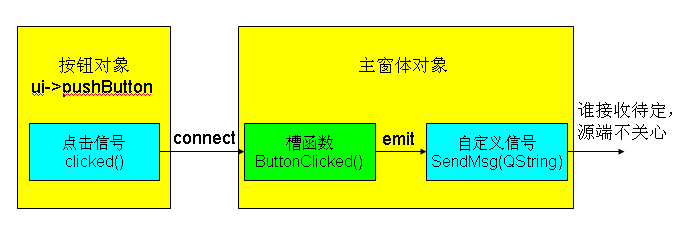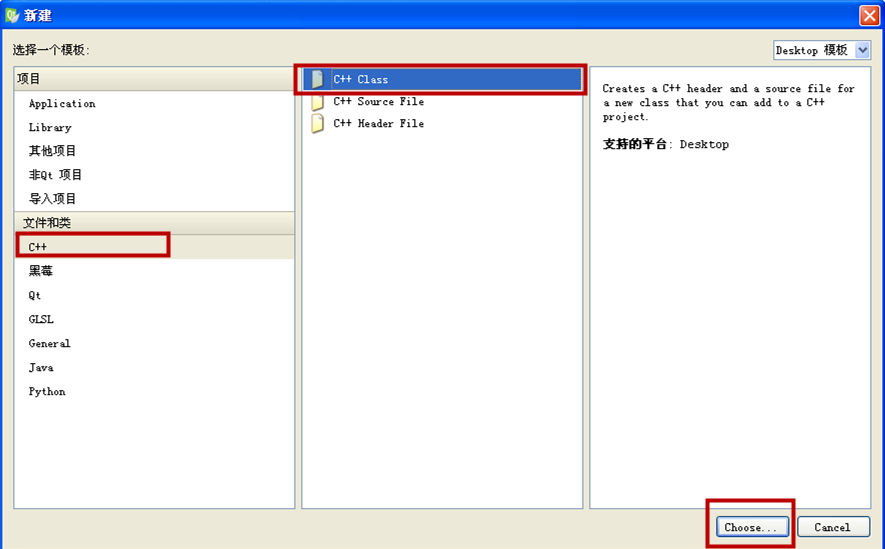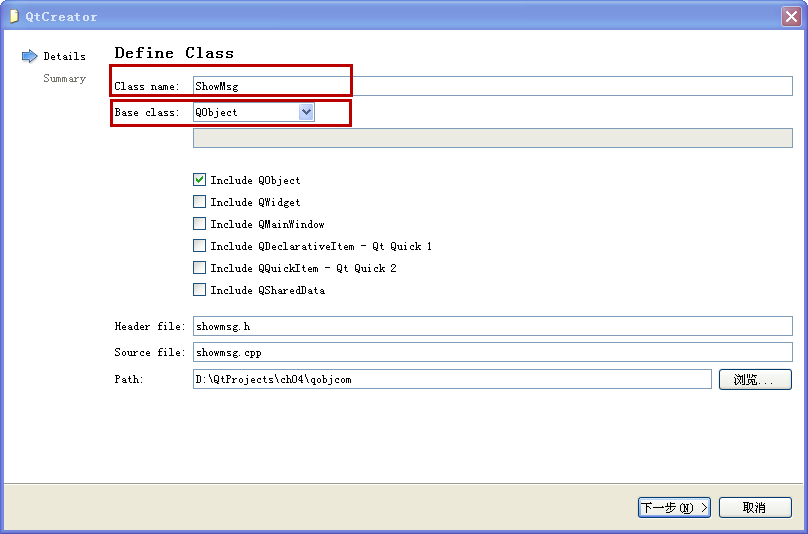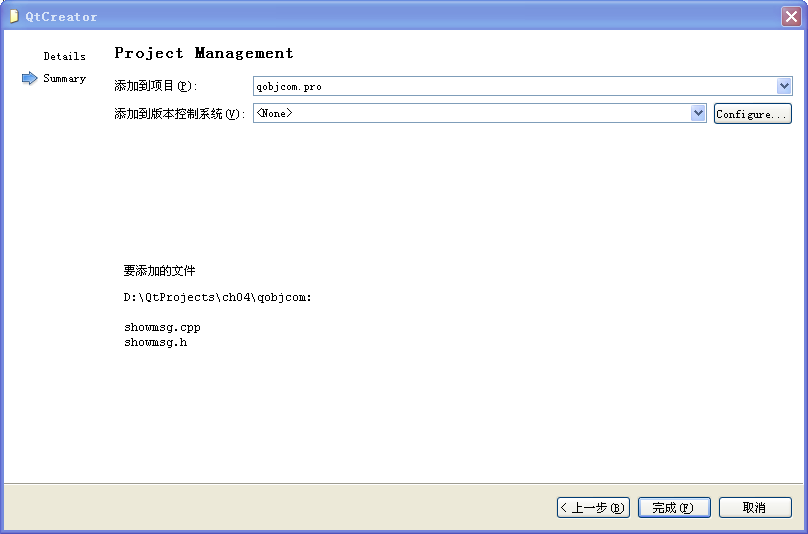#ifndef SHOWMSG_H
`#define SHOWMSG_H`

`#include <QObject>`

`class ShowMsg : public QObject`
`{`
`    Q_OBJECT`
`public:`
`    explicit ShowMsg(QObject *parent = 0);`
`    ~ShowMsg();`

`signals:`

`public slots:`
`    //接收 SendMsg 信号的槽函数`
`    void RecvMsg(QString str);`
`};`

`#endif // SHOWMSG_H`
RecvMsg 槽函数声明的参数类型和返回类型要与 SendMsg 信号保持一致，所以参数是 QString，返回 void。

#include "showmsg.h"
`#include <QMessageBox>`

`ShowMsg::ShowMsg(QObject *parent) : QObject(parent)`
`{`

`}`

`ShowMsg::~ShowMsg()`
`{`

`}`

`//str 就是从信号里发过来的字符串`
`void ShowMsg::RecvMsg(QString str)`
`{`
`    QMessageBox::information(NULL, tr("Show"), str);`
`}`

#include "widget.h"
`#include <QApplication>`
`#include "showmsg.h"`

`int main(int argc, char *argv[])`
`{`
`    QApplication a(argc, argv);`
`    Widget w;   //①主窗体对象，内部会发送 SendMsg 信号`
`    ShowMsg s;  //②接收端对象，有槽函数 RecvMsg`
`    //③关联，信号里的字符串参数会自动传递给槽函数`
`    QObject::connect(&w, SIGNAL(SendMsg(QString)), &s, SLOT(RecvMsg(QString)));`

`    //显示主界面`
`    w.show();`

`    return a.exec();`
`}`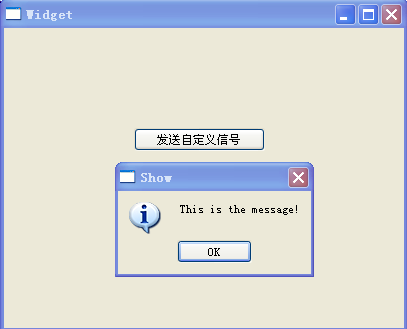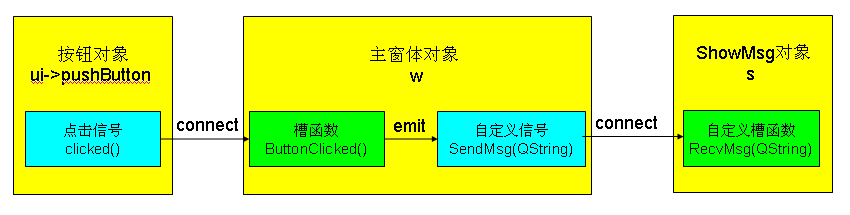4.3.3 信号关联到信号示例

①项目名称 signalsconn，创建路径 D:\QtProjects\ch04，点击下一步；
②套件选择里面选择全部套件，点击下一步；
③基类选择 QWidget，点击下一步；
④项目管理不修改，点击完成。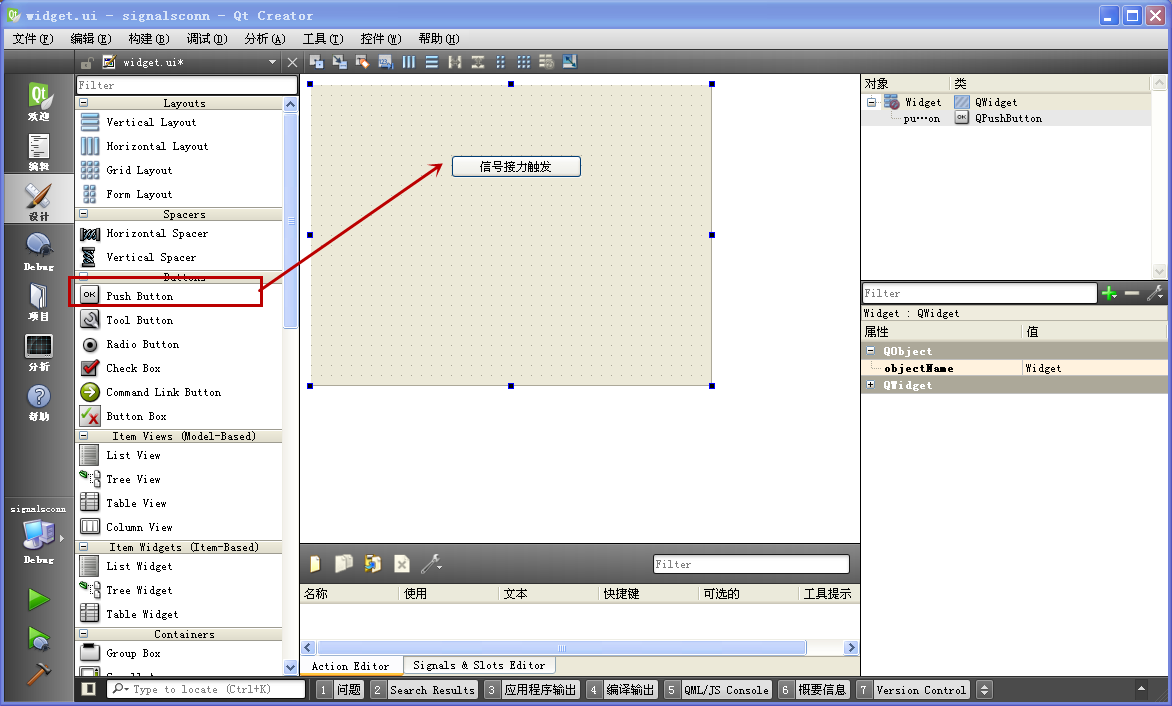#ifndef WIDGET_H
`#define WIDGET_H`

`#include <QWidget>`

`namespace Ui {`
`class Widget;`
`}`

`class Widget : public QWidget`
`{`
`    Q_OBJECT`

`public:`
`    explicit Widget(QWidget *parent = 0);`
`    ~Widget();`

`signals:    //只添加一个信号，不需要按钮对应的槽函数`
`    void SendVoid();`

`private:`
`    Ui::Widget *ui;`
`};`

`#endif // WIDGET_H`

#include "widget.h"
`#include "ui_widget.h"`

`Widget::Widget(QWidget *parent) :`
`    QWidget(parent),`
`    ui(new Ui::Widget)`
`{`
`    ui->setupUi(this);`

`    //关联按钮信号到自定义的信号`
`    connect(ui->pushButton, SIGNAL(clicked()), this, SIGNAL(SendVoid()));`
`}`

`Widget::~Widget()`
`{`
`    delete ui;`
`}`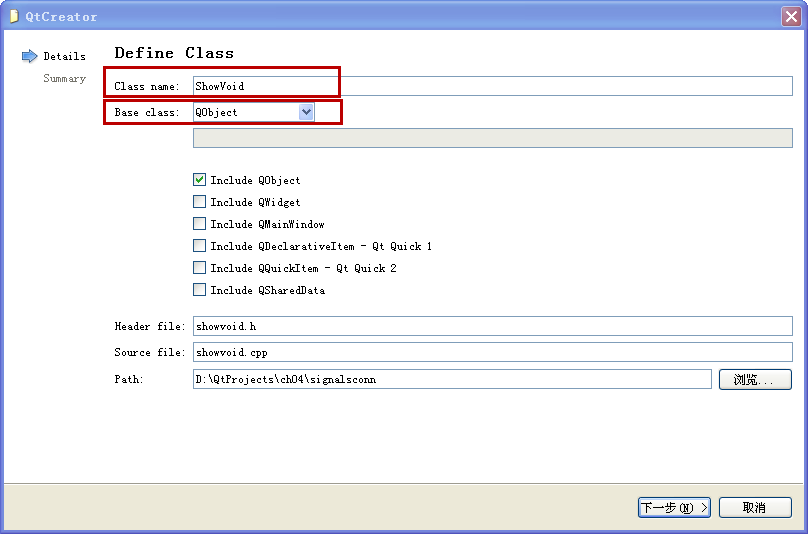#ifndef SHOWVOID_H
`#define SHOWVOID_H`

`#include <QObject>`

`class ShowVoid : public QObject`
`{`
`    Q_OBJECT`
`public:`
`    explicit ShowVoid(QObject *parent = 0);`
`    ~ShowVoid();`

`signals:`

`public slots:`
`    //接收 SendVoid() 信号的槽函数`
`    void RecvVoid();`
`};`

`#endif // SHOWVOID_H`

#include "showvoid.h"
`#include <QMessageBox>`

`ShowVoid::ShowVoid(QObject *parent) : QObject(parent)`
`{`

`}`

`ShowVoid::~ShowVoid()`
`{`

`}`
`//槽函数，弹窗`
`void ShowVoid::RecvVoid()`
`{`
`    QMessageBox::information(NULL, tr("Show"), tr("Just void."));`
`}`

#include "widget.h"
`#include <QApplication>`
`#include "showvoid.h"`

`int main(int argc, char *argv[])`
`{`
`    QApplication a(argc, argv);`
`    Widget w;   //①源头对象，内部发送信号 SendVoid()`
`    ShowVoid s; //②接收对象，有对应槽函数　RecvVoid()`
`    //③关联源头的信号和接收端的槽函数`
`    QObject::connect(&w, SIGNAL(SendVoid()), &s, SLOT(RecvVoid()));`

`    //显示主界面`
`    w.show();`

`    return a.exec();`
`}`